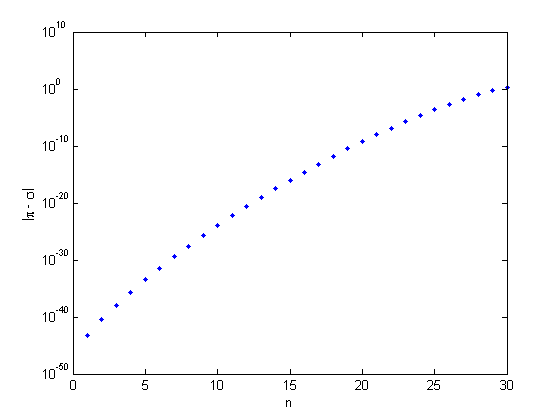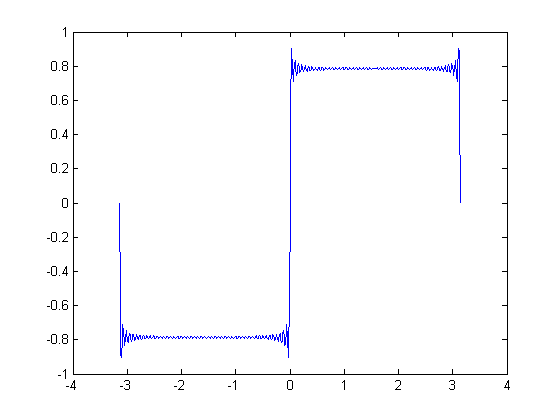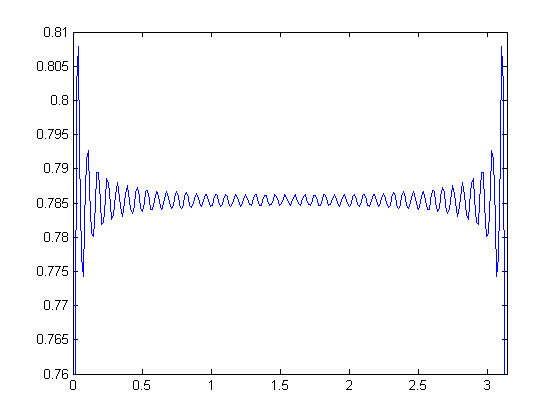# Surprising SVD, Square Waves, and Pi

I am surprised when many of the singular values of a nonsymmetric variant of the Hilbert matrix turn out to be nearly equal to $\pi$. The explanation involves the Fourier series for a square wave.

### Contents

#### The Nonsymmetric Hilbert Matrix

My very first project in matrix computation involved the Hilbert matrix. It had been introduced in 1894 by the famous mathematician David Hilbert as what we would today call the coefficient matrix in the normal equations for least squares approximations by polynomials with their monomial basis. The elements of an $n$ -by- $n$ Hilbert matrix are

$$a_{i,j} = \frac{1}{i+j-1}, \ \ i,j = 1,...,n$$

Some years ago I wanted a nonsymmetric test matrix, so I changed the plus sign in the denominator to a minus sign, but those elements blew up along the subdiagonal, so I also changed the -1 to +1/2.

$$a_{i,j} = \frac{1}{i-j+1/2}, \ \ i,j = 1,...,n$$

I refer to this as the nonsymmetric Hilbert Matrix. Here is the 5-by-5 with format rat.

   format rat
n = 5;
[I,J] = ndgrid(1:n);
A = 1./(I-J+1/2)

A =

2             -2             -2/3           -2/5           -2/7
2/3            2             -2             -2/3           -2/5
2/5            2/3            2             -2             -2/3
2/7            2/5            2/3            2             -2
2/9            2/7            2/5            2/3            2



#### Singular Values

I was amazed when I happened to compute the singular values. Here are all of the singular values of the 30-by-30 nonsymmetric Hilbert matrix.

   format long
n = 30;
[I,J] = ndgrid(1:n);
A = 1./(I-J+1/2);
sigma = svd(A)

sigma =

3.141592653589795
3.141592653589794
3.141592653589794
3.141592653589794
3.141592653589794
3.141592653589794
3.141592653589793
3.141592653589793
3.141592653589793
3.141592653589793
3.141592653589793
3.141592653589792
3.141592653589792
3.141592653589792
3.141592653589791
3.141592653589779
3.141592653589737
3.141592653588278
3.141592653559363
3.141592653039148
3.141592644613932
3.141592521935767
3.141590919627153
3.141572215205717
3.141378147613475
3.139604197477250
3.125546289482884
3.032461817019588
2.564498174433655
1.105241141304968



Incredibly, half of them are equal to pi to the full length of our long format, that's 16 digits. And all but the last few are equal to pi to at least a couple of digits. How did that happen?

#### High Precision Calculation

Let's do the 30-by-30 SVD again, this time using the Symbolic Toolbox with 100-digit arithmetic. The computation takes about a minute and a half on my machine.

   tic
digits(100)
n = 30;
[I,J] = ndgrid(sym(1:n));
A = 1./(I-J+1/2);
sigma = svd(A);
toc

Elapsed time is 83.939032 seconds.


Here is the first singular value, to 100 digits.

   sigma1 = sigma(1)


sigma1 =

3.141592653589793238462643383279502884197169352107009745551549597527462440105298598090594765999823398



Here is a logarithmic plot of the difference between the singular values and $\pi$.

   semilogy(abs(pi - sigma),'.')
xlabel('n')
ylabel('|\pi - \sigma|');The first singular value equals $\pi$ to 44 digits and, as our double precision computation predicted, fifteen of the thirty singular values equal $\pi$ to at least 15 digits.

#### Seymour Parter

Seymour Parter was a visiting professor at Stanford when I was a grad student there in the early 1960s. I took an individual reading course under his supervision. He went from Stanford to the University of Wisconsin, where he spent the rest of his career as a Professor of Mathematics and Computer Sciences. He was President of SIAM in 1981 and '82.

In 1985, the Second SIAM Conference on Linear Algebra was held at North Carolina State University in Raleigh. I demonstrated MATLAB during the coffee breaks. We didn't really have a booth, just a desk or table. I used this example in my demonstration and asked conference attendees if they could explain the appearance of $\pi$. Seymour said that he could. He had written two papers twenty years earlier about eigenvalues of Toeplitz matrices that were relevant. He soon produced another paper, referenced below, that explains the behavior of the singular values of my nonsymmetric Hilbert matrix. It is related to a result from 1920 by the famous Hungarian mathematician Gabor Szego.

#### Toeplitz Matrices

Our matrix $A$ is constant along its diagonals, so it is a Toeplitz matrix. So is the $2n$ -by- $2n$ matrix $B$ constructed from $A$ by the MATLAB array operation

Z = zeros(size(A));
B = -i*[Z A; -A' 0];

The matrix $B$ is both Hermitian and Toeplitz. Its eigenvalues are real and occur in plus/minus pairs. The absolute values of the eigenvalues of $B$ are the singular values of $A$, each repeated twice.

Szego's 1920 theorem says that the eigenvalues of the Toeplitz matrix formed from the coefficients in the Fourier expansion of a function converge to the max and min of that function.

Parter realized two important facts. First, that he could analyze the singular values of my nonsymmetric matrix $A$ by looking at the eigenvalues of the Hermitian matrix $B$. And second, that the elements of $A$ and $B$ are the reciprocals of odd integers, which are the coefficients of the Fourier expansion of a square wave. So Szego's 1920 theorem implies that the largest eigenvalues of $B$ and hence the largest singular values of $A$ converge to the maximum of that square wave.

#### Square Wave

There is already a hint of what is going on in an old MATLAB demo named xfourier. The first line of xfourier says

This example shows (graphically) how the Fourier series expansion for a square wave is made up of a sum of odd harmonics.

The core of xfourier is a code fragment similar to this.

   t = -pi:pi/256:pi;
y = zeros(size(t));
for k = 1:2:99
y = y + sin(k*t)/k;
end
plot(t,y)The plot shows two things. First you notice the overshoot at the ends of the intervals known as the Gibbs phenomenon. But more important for our purposes here is the convergence in the interior of the intervals to values near plus and minus 0.8.

Let's use more terms and take a closer look.

   t = -pi:pi/256:pi;
y = zeros(size(t));
for k = 1:2:599
y = y + sin(k*t)/k;
end
plot(t,y)
axis([0 pi .76 .81])We find the sum is converging pointwise to a value near 0.7854. The fragment code is approximating the Fourier sine expansion

$$s(t) = \sum_{odd\ k}\frac{\sin{kt}}{k}$$

The coefficients are the reciprocals of odd integers, which are the same as the elements of the nonsymmetric Hilbert matrix.

The Fourier series converges pointwise to $\pm\pi/4$. If we trace this fact back through Parter's analysis and Szego's theorem, we have an explanation for the fact that the largest singular values of the nonsymmetric Hilbert matrix are converging to $\pi$.

#### E. E. Tyrtyshnikov

Evgenij E. Tyrtyshnikov is a Professor and Deputy Director of the Institute of Numerical Mathematics of the Russian Academy of Sciences in Moscow. In 1991 he studied the behavior of the small singular values of this matrix. And in 1992 he considered the matrices where the 1/2 is replaced by any noninteger value.

#### References

Seymour V. Parter, On the distribution of the singular values of Toeplitz matrices, Linear Algebra and its Applications 80, 1986, 115-130, <http://www.sciencedirect.com/science/article/pii/0024379586902806>

Evgenij E. Tyrtyshnikov, Cauchy-Toeplitz matrices and some applications, Linear Algebra and its Applications 149, 1991, 1-18, <http://www.sciencedirect.com/science/article/pii/002437959190321M>

Evgenij E. Tyrtyshnikov, Singular values of cauchy-toeplitz matrices, Linear Algebra and its Applications 161, 1992, 99-116, <http://www.sciencedirect.com/science/article/pii/002437959290007W>

Published with MATLAB® R2014a

|

### コメント

コメントを残すには、ここ をクリックして MathWorks アカウントにサインインするか新しい MathWorks アカウントを作成します。